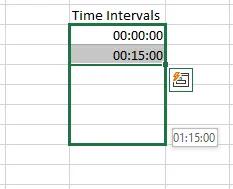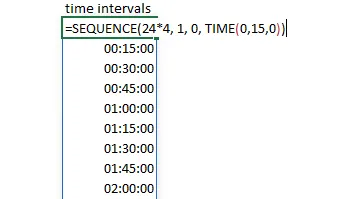# How to generate time intervals in Excel

Sometimes it is necessary to generate time intervals in Excel to make sure that there are all of them. It is an easy and flexible way to do this by using the relatively new Excel function SEQUENCE.

## Generate time intervals in Excel

Of course, there is possible to write two sequential entries, like in the picture below, and use a fill handle. It is an acceptable solution in an appropriate situation.If you want more freedom and flexible results, it is also possible with the Excel function SEQUENCE.

For example, it is necessary to get the date and time sequence with 15-minute intervals. Firstly, here is how to generate only 15-minute time intervals in Excel.

`=SEQUENCE(24*4, 1, 0, TIME(0,15,0))`The first argument is the calculation gives us how many 15-minute intervals are in one day, the second is columns with this sequence, the third is the beginning, and the last one is the step.

By using the same technique, you can create any other time interval, for example, 10-minute intervals, 30-minute intervals in Excel, etc.

## Date and time intervals in Excel

You can do necessary date calculations based on today’s date and add that to SEQUENCE like this. Add that as the beginning of the sequence and adjust the format of the result.

`=SEQUENCE(24*4, 1, TODAY(), TIME(0,15,0))`

## Determine the period for the sequence in Excel

If you have a sequence with time or date and time combination, it might be necessary to determine a specific period. It depends on how you like to look at this. You might want to look at the beginning of the time interval or at the end of the time interval.

To eliminate the need to write repeatedly same thing you can use variables by using the Excel function LET. Here is more about that.

By using the function LET, I created a variable Time_Sequence with a previously used date and time list. After creating a variable, it is used in the FILTER function, where it is a filter applied to get the DateTime sequence from eight in the morning to nine in the evening.

```=LET(
Time_Sequence,
SEQUENCE(24*4, 1, TODAY(), TIME(0,15,0)),

FILTER(Time_Sequence, (HOUR(Time_Sequence) >= 8) * (HOUR(Time_Sequence) < 21)))```